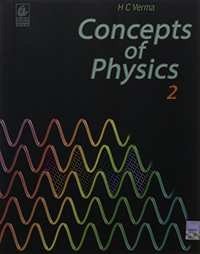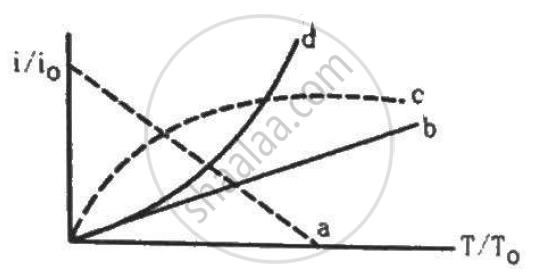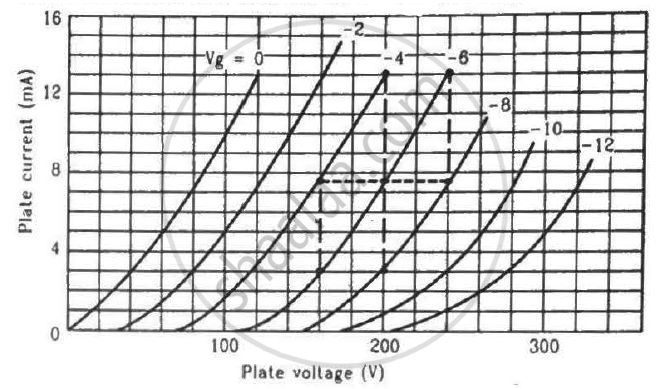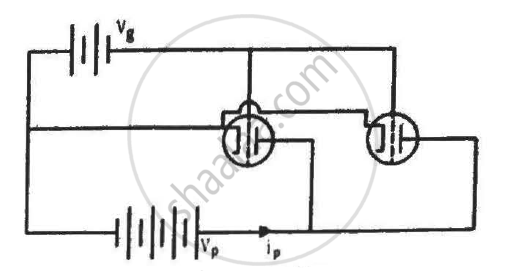Share

# HC Verma solutions for Class 12 Concepts of Physics Vol. 2 chapter 19 - Electric Current through Gases [Latest edition]

Textbook page

#### Chapters## Chapter 19: Electric Current through Gases

#### HC Verma solutions for Class 12 Concepts of Physics Vol. 2 Chapter 19 Electric Current through Gases [Page 351]

Q 1 | Page 351

Why is conducting easier in gases if the pressure is low? Will the conduction continue to improve if the pressure is made as low as nearly zero?

Q 2 | Page 351

An AC source is connected to a diode and a resistor in series. Is the current thorough the resistor AC or DC?

Q 3 | Page 351

How will the thermionic current vary if the filament current is increased?

Q 4 | Page 351

Would you prefer a material with a high melting point or a low melting point to be used as a cathode in a diode?

Q 5 | Page 351

Would you prefer a material with a high work-function or a low work-function to be used as a cathode in a diode?

Q 6 | Page 351

An isolated metal sphere is heated to a high temperature. Will it become positively charged due to thermionic emission?

Q 7 | Page 351

A diode value is connected to a battery and a load resistance. The filament is heated, so that a constant current is obtained in the circuit. As the cathode continuously emits electrons, does it become more and more positively charged?

Q 8 | Page 351

Why does thermionic emission not take place in non-conductors?

Q 9 | Page 351

The cathode of a diode valve is replaced by another cathode of double the surface area. Keeping the voltage and temperature conditions the same, will the place current decrease, increase or remain the same?

Q 10 | Page 351

Why is the linear portion of the triode characteristic chosen to operate the triode as an amplifier?

#### HC Verma solutions for Class 12 Concepts of Physics Vol. 2 Chapter 19 Electric Current through Gases [Pages 351 - 352]

Q 1 | Page 351

Cathode rays constitute a stream of _______________ .

• electrons

• protons

• positive ions

• negative ions

Q 2 | Page 351

Cathode rays are passing through a discharge tube. In the tube, there is ______________ .

• an electric field but no magnetic field

• a magnetic field but no electric field

• an electric as well as a magnetic field

• neither an electric nor a magnetic field

Q 3 | Page 352

Let i0 be the thermionic current from a metal surface when the absolute temperature of the surface is T0. The temperature is slowly increased and the thermionic current is measured as a function of temperature. Which of the following plots may represent the variation in (i/i0) against (T/T0)?• a

• b

• c

• d

Q 4 | Page 352

When the diode shows saturated current, dynamic place resistance is _____________ .

• zero

• infinity

• indeterminate

• different for different diodes

Q 5 | Page 352

The anode of a thermionic diode is connected to the negative terminal of a battery and the cathode to its positive terminal.

• No appreciable current will pass through the diode.

• A large current will pass through the diode from the anode to the cathode.

• A large current will pass through the diode from the cathode to the anode.

• The diode will be damaged.

Q 6 | Page 352

A diode, a resistor and a 50 Hz AC source are connected in series. The number of current pulses per second through the resistor is __________ .

• 25

• 50

• 100

• 200

Q 7 | Page 352

A triode is operated in the linear region of its characteristics. If the plate voltage is slightly increased, the dynamic plate resistance will __________ .

• increase

• decrease

• remain almost the same

• become zero

Q 8 | Page 352

The plate current in a triode value is maximum when the potential of the grid is ______________ .

• positive

• zero

• negative

• non-positive

Q 9 | Page 352

The amplification factor of a triode operating in the linear region depends strongly on ____________ .

• the temperature of the cathode

• the plate potential

• the grid potential

• the separations of the grid from the cathode and the anode

#### HC Verma solutions for Class 12 Concepts of Physics Vol. 2 Chapter 19 Electric Current through Gases [Page 352]

Q 1 | Page 352

Electric conduction takes place in a discharge tube due to the movement of _____________ .
(a) positive ions
(b) negative ions
(c) electrons
(d) protons

Q 2 | Page 352

Which of the following is true for a cathode ray?
(a) It travels in a straight line.
(b) It emits an X-ray when it strikes a metal.
(c) It is an electromagnetic wave.
(d) It is not deflected by a magnetic field.

Q 3 | Page 352

Due to the space charge in a diode valve, _____________ .

• the plate current decreases

• the plate voltage increases

• the rate of emission of thermions increases

• the saturation current increases

Q 4 | Page 352

The saturation current in a triode valve can be changed by changing _____________ .

• the grid voltage

• the plate voltage

• the separation between the grid and the cathode

• the temperature of the cathode

Q 5 | Page 352

Mark the correct options.
(a) A diode valve can be used as a rectifier.
(b) A triode valve can be used as a rectifier.
(c) A diode valve can be used as an amplifier.
(d) A triode valve can be used as an amplifier.

Q 6 | Page 352

The plate current in a diode is zero. It is possible that _____________ .

(a) the plate voltage is zero
(b) the plate voltage is slightly negative
(c) the plate voltage is slightly positive
(d) the temperature of the filament is low

Q 7 | Page 352

The plate current in a triode valve is zero. The temperature of the filament is high. It is possible that ____________ .

(a) Vg > 0, Vp > 0
(b) Vg > 0, Vp < 0
(c) Vg < 0, Vp > 0
(d) Vg < 0, Vp < 0

#### HC Verma solutions for Class 12 Concepts of Physics Vol. 2 Chapter 19 Electric Current through Gases [Pages 352 - 354]

Q 1 | Page 352

A discharge tube contains helium at low pressure. A large potential difference is applied across the tube. Consider a helium atom that has just been ionised due to the detachment of an atomic electron. Find the ratio of the distance travelled by the free electron to that by the positive ion in a short time dt after ionisation.

Q 2 | Page 352

A molecule of a gas, filled in a discharge tube, gets ionised when an electron is detached from it. An electric field of 5.0 kV m−1 exists in the vicinity of the event. (a) Find the distance travelled by the free electron in 1 µs, assuming there's no collision. (b) If the mean free path of the electron is 1.0 mm, estimate the time of transit of the free electron between successive collisions.

Q 3 | Page 353

The mean free path of electrons in a gas in a discharge tube is inversely proportional to the pressure inside it. The Crookes dark space occupies half the length of the discharge tube when the pressure is 0.02 mm of mercury. Estimate the pressure at which the dark space will fill the whole tube.

Q 4 | Page 353

Two discharge tubes have identical material structures and the same gas is filled in them. The length of one tube is 10 cm and that of the other tube is 20 cm. Sparking starts in both the tubes when the potential difference between the cathode and the anode is 100 V. If the pressure in the shorter tube is 1.0 mm of mercury, what is the pressure in the longer tube?

Q 5 | Page 353

Calculate n(T)/n(1000 K) for tungsten emitter at T = 300 K, 2000 K and 3000 K, where n(T) represents the number of thermions emitted per second by the surface at temperature T. Work function of tungsten is 4.52 eV.

Q 6 | Page 353

The saturation current from a thoriated-tungsten cathode at 2000 K is 100 mA. What will be the saturation current for a pure-tungsten cathode of the same surface area operating at the same temperature? The constant A in the Richardson-Dushman equation is 60 × 104 Am −2 K−2 for pure tungsten and 3.0 × 104 Am −2 k−2 for thoriated tungsten. The work function of pure tungsten is 4.5 eV and that of thoriated tungsten is 2.6 eV.

Q 7 | Page 353

A tungsten cathode and a thoriated-tungsten cathode have the same geometric dimensions and are operated at the same temperature. The thoriated-tungsten cathode gives 5000 times more current than the other cathode. The constant A in the Richardson−Dushman equation is 60 × 104 Am −2 K−2 for pure tungsten and 3.0 × 104 Am −2 k−2 for thoriated tungsten. The work function of pure tungsten is 4.5 eV and that of thoriated tungsten is 2.6 eV. Find the operating temperature.

Q 8 | Page 353

If the temperature of a tungsten filament is raised from 2000 K to 2010 K, by what factor does the emission current change? Work function of tungsten is 4.5 eV.

Q 9 | Page 353

The constant A in the Richardson−Dushman equation for tungsten is 60 × 104 A m−2K−2. The work function of tungsten is 4.5 eV. A tungsten cathode with a surface area 2.0 × 10−5 m2 is heated by a 24 W electric heater. In steady state, the heat radiated by the heater and the cathode equals the energy input by the heater and the temperature becomes constant. Assuming that the cathode radiates like a blackbody, calculate the saturation current due to thermions. Take Stefan's Constant = 6 × 10−8 W m−2 K−1. Assume that the thermions take only a small fraction of the heat supplied.

Q 10 | Page 353

A plate current of 10 mA is obtained when 60 volts are applied across a diode tube. Assuming the Langmuir-Child relation $i_p \infty V_p^{3/2}$ to hold, find the dynamic resistance rp in this operating condition.

Q 11 | Page 353

The plate current in a diode is 20 mA when the plate voltage is 50 V or 60 V. What will be the current if the plate voltage is 70 V?

Q 12 | Page 353

The power delivered in the plate circular of a diode is 1.0 W when the plate voltage is 36 V. Find the power delivered if the plate voltage is increased to 49 V. Assume Langmuir-Child equation to hold.

Q 13 | Page 353

A triode value operates at Vp = 225 V and Vg = −0.5 V.
The plate current remains unchanged if the plate voltage is increased to 250 V and the grid voltage is decreased to −2.5 V. Calculate the amplification factor.

Q 14 | Page 353

Calculate the amplification factor of a triode valve that has plate resistance of 2 kΩ and transconductance of 2 millimho.

Q 15 | Page 353

The dynamic plate resistance of a triode value is 10 kΩ. Find the change in the plate current if the plate voltage is changed from 200 V to 220 V.

Q 16 | Page 353

Find the values of rp, µ and gm of a triode operating at plate voltage 200 V and grid voltage −6. The plate characteristics are shown in the figure.Q 17 | Page 353

The plate resistance of a triode is 8 kΩ and the transconductance is 2.5 millimho. (a) If the plate voltage is increased by 48 V and the grid voltage is kept constant, what will be the increase in the plate current? (b) With plate voltage kept constant at this increased value, by how much should the grid voltage be decreased in order to bring the plate current back to its initial value?

Q 18 | Page 353

The plate resistance and amplification factor of a triode are 10kΩ and 20. The tube is operated at plate voltage 250 V and grid voltage −7.5 V. The plate current is 10 mA. (a) To what value should the grid voltage be changed so as to increase the plate current to 15 mA? (b) To what value should the plate voltage be changed to take the plate current back to 10 mA?

Q 19 | Page 353

The plate current, plate voltage and grid voltage of a 6F6 triode tube are related as ip = 41 (Vp + 7 Vg)1.41

Here, Vp and Vg are in volts and ip in microamperes.
The tube is operated at Vp = 250 V, Vg = −20 V. Calculate (a) the tube current, (b) the plate resistance, (c) the mutual conductance and (d) the amplification factor.

Q 20 | Page 353

The plate current in a triode can be written as $i_p = k \left( V_g + \frac{V_p}{\mu} \right)^{3/2}$ Show that the mutual conductance is proportional to the cube root of the plate current.

Q 21 | Page 353

A triode has mutual conductance of 2.0 millimho and plate resistance of 20 kΩ. It is desired to amplify a signal by a factor of 30. What load resistance should be added in the circuit?

Q 22 | Page 354

The gain factor of an amplifier in increased from 10 to 12 as the load resistance is changed from 4 kΩ to 8 kΩ. Calculate (a) the amplification factor and (b) the plate resistance.

Q 23 | Page 354

The figure shows two identical triode tubes connected in parallel. The anodes are connected together and the cathodes are connected together. Show that the equivalent plate resistance is half the individual plate resistance, the equivalent mutual conductance is double the individual mutual conductance and the equivalent amplification factor is the same as the individual amplification factor.## Chapter 19: Electric Current through Gases## HC Verma solutions for Class 12 Concepts of Physics Vol. 2 chapter 19 - Electric Current through Gases

HC Verma solutions for Class 12 Concepts of Physics Vol. 2 chapter 19 (Electric Current through Gases) include all questions with solution and detail explanation. This will clear students doubts about any question and improve application skills while preparing for board exams. The detailed, step-by-step solutions will help you understand the concepts better and clear your confusions, if any. Shaalaa.com has the CBSE Class 12 Concepts of Physics Vol. 2 solutions in a manner that help students grasp basic concepts better and faster.

Further, we at Shaalaa.com provide such solutions so that students can prepare for written exams. HC Verma textbook solutions can be a core help for self-study and acts as a perfect self-help guidance for students.

Concepts covered in Class 12 Concepts of Physics Vol. 2 chapter 19 Electric Current through Gases are Einstein’S Photoelectric Equation: Energy Quantum of Radiation, Particle Nature of Light: the Photon, Photoelectric Effect and Wave Theory of Light, Experimental Study of Photoelectric Effect, Einstein’s Equation - Particle Nature of Light, Electron Emission, Davisson-Germer Experiment, de-Broglie Relation, Wave Nature of Matter, Photoelectric Effect - Hallwachs’ and Lenard’S Observations, Photoelectric Effect - Hertz’S Observations, Dual Nature of Radiation, Concept of Semiconductor Electronics: Materials, Devices and Simple Circuits, Integrated Circuits, Feedback Amplifier and Transistor Oscillator, Transistor as a Device, Basic Transistor Circuit Configurations and Transistor Characteristics, Application of Junction Diode as a Rectifier, p-n Junction, Intrinsic Semiconductor, Classification of Metals, Conductors and Semiconductors, Extrinsic Semiconductor, Transistor Action, Transistor: Structure and Action, Digital Electronics and Logic Gates, Transistor as an Amplifier (Ce-configuration), Transistor and Characteristics of a Transistor, Semiconductor Diode, Zener Diode as a Voltage Regulator, Special Purpose P-n Junction Diodes, Diode as a Rectifier, Energy Bands in Conductors, Semiconductors and Insulators, Triode.

Using HC Verma Class 12 solutions Electric Current through Gases exercise by students are an easy way to prepare for the exams, as they involve solutions arranged chapter-wise also page wise. The questions involved in HC Verma Solutions are important questions that can be asked in the final exam. Maximum students of CBSE Class 12 prefer HC Verma Textbook Solutions to score more in exam.

Get the free view of chapter 19 Electric Current through Gases Class 12 extra questions for Class 12 Concepts of Physics Vol. 2 and can use Shaalaa.com to keep it handy for your exam preparation

S